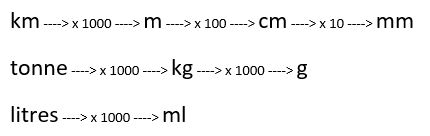# Conversion of Units

There are few easier calculations in maths than converting metric units, but the number of errors that are made always astounds me. Most of the time it is down to laziness, not reading the question or moving the digits in the wrong direction.

The basic premise, now that we have safely adopted the metric system and all mathematics has become standardised and straightforward, is that the figures can be kept in the same order and simply moved along to the right or the left of the decimal point, as in the previous walkthroughs about multiplication / division and place value.

Children are automatically able to convert pounds and pence into just pounds or just pence; they do this so much and from an early age, that when they get asked it takes little thought. 435p is clearly the same as £4.35. However, ask them to convert 435cm into metres and sometimes the thought processes take a totally different tack, even though the calculations are identical.

Put simply, here are the calculations that need to be carried out, step by step.The same applies going the other way but the multiplications are replaced by divisions.

Therefore, to convert km into mm, we must:

multiply the number of km by 1000 to get a number of metres

multiply this number by 100 to change it to cm

multiply this number by 10 to convert it to mm.

Overall, we have multiplied by 1000 x 100 x 10 = 1 000 000

Questions may take the form of 'which is greater - 2330 cm or 0.233 km?'

To compare two things they need to be in the same units, so to get from 0.233 km to cm, we multiply by 1000 then by 100 (or by 100 000 in one go) and find that 0.233km = 23300 cm. Therefore, it is greater than 2330 cm.